# Evaluating Model Performance

## Regression Performance

Evaluating performance of a regression model requires a different approach and different metrics than are used to evaluate classification models. Regression models estimate continuous values; therefore, regression performance metrics quantify how close model predictions are to actual (true) values.

The following are some commonly used regression performance metrics.

#### Coefficient of Discrimination, R-squared (R2)

R2 is an indicator of how well a regression model fits the data. It represents the extent to which the variation of the dependent variable is predictable by the model.

For example, an R2 value of 1 indicates that the input variables in the model (such as sales history and marketing engagement for customer attrition) are able to explain all of the variation observed in the output (such as number of customers who unsubscribed). If a model has a low R2 value, it may indicate that other inputs should be added to improve accuracy.

Mathematically, R2 is defined as: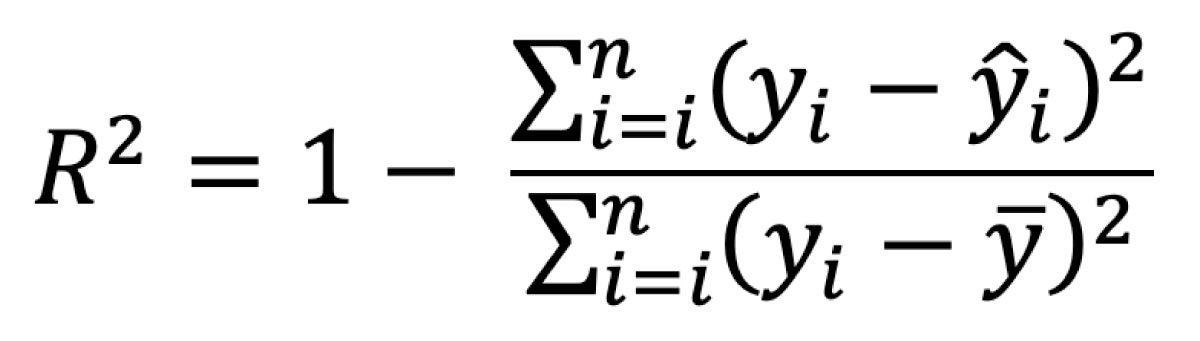where n is the total number of evaluated samples, yi is the ith observed output, ŷi is the ith predicted output, and ȳ is the mean observed output. The quantity (yi – ŷi) can also be referred to as the prediction error, denoted êi.

Let’s consider a simple regression model that is trained to forecast monthly sales at a company. The following table illustrates the concept.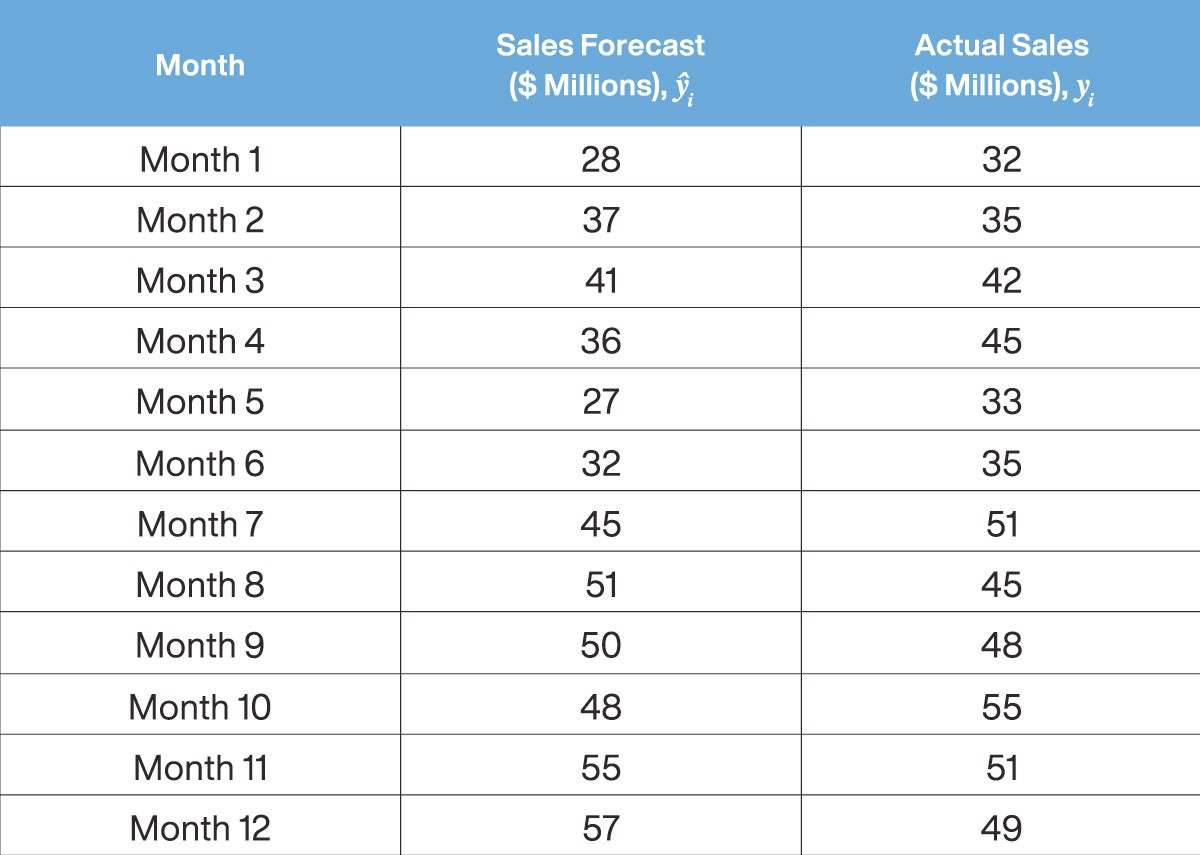Table 1 Example of a simple sales forecasting model

A data scientist may want to compare the model’s performance relative to actuals (for instance, over the last year). A data scientist using R2 to estimate model performance would perform the calculation described in the following table. The R2 value for this sales forecasting model is 0.7.Table 2 Calculation of R2 for the Simple Sales Forecasting Model

#### Mean Absolute Error (MAE)

MAE measures the absolute error between predicted and observed values. For example, an MAE value of 0 indicates there is no difference between predicted values and observed values. In practice, MAE is a popular error metric because it is both intuitive and easy to compute.

Mathematically, MAE is defined as: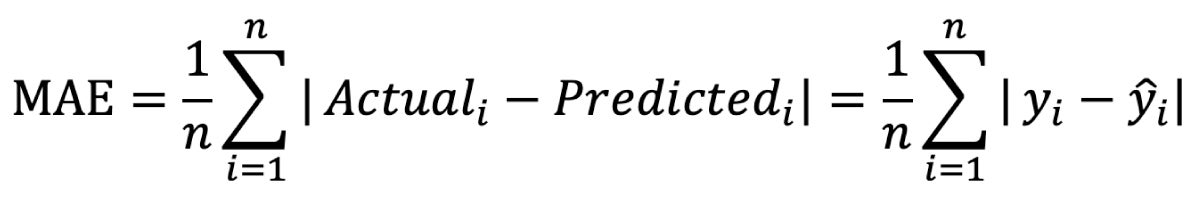where n is the total number of evaluated samples, yi is the ith observed (actual) output, and ŷi is the ith predicted output.

#### Mean Absolute Percent Error (MAPE)

MAPE measures the average absolute percent error of predicted values versus observed values. Normalizing for the relative magnitude of observed values reduces skew in the reporting metric so it is not overly weighted by large magnitude values. MAPE is commonly used to evaluate the performance of forecasting models.

Mathematically, MAPE is defined as: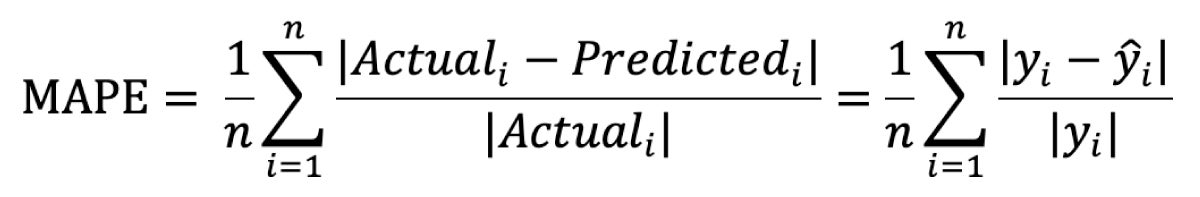where n is the total number of evaluated samples, yi is the ith observed (actual) output, and ŷi is the ith predicted output.

#### Root Mean Square Error (RMSE)

RMSE is a quadratic measure of the error between predicted and observed values. It is similar to MAE as a way to measure the magnitude of model error, but because RMSE averages the square of errors, it provides a higher weight to large magnitude errors. RMSE is a commonly used metric in business problems where higher magnitude errors have a higher consequence – like predicting item sales prices, where high-priced items matter more for bottom-line business goals. However, this also may result in over-sensitivity to outliers.

Mathematically, RMSE is defined as: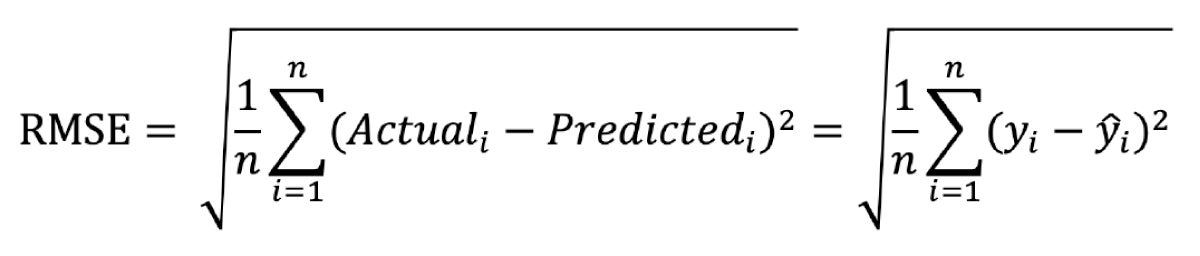where n is the total number of evaluated samples, yi is the ith observed (actual) output, and ŷi is the ith predicted output.

We can now compute MAE, MAPE, and RMSE for the same monthly sales forecasting example, as outlined in the following table.Table 3 Calculation of MAE, MAPE, and RMSE for the Simple Sales Forecasting Model

As seen in Tables 2 and 3, the R2 (0.7) and MAPE (0.11) regression metrics provide a normalized relative sense of model performance. A “perfect” model would have an R2 value of 1. The MAPE metric provides an intuitive sense of the average percentage deviation of model predictions from actuals. In this case, the model is approximately 11 percent “off.”

The MAE (4.8) and RMSE (5.4) metrics provide a non-normalized, absolute sense of model performance in the predicted unit (in this case millions of dollars). MAE provides a sense of the average absolute value of the forecast’s deviation from actuals. Finally, RMSE provides a “root-mean-square” version of the forecasts’ average deviations from actuals.